GeeksforGeeks App
Open AppBrowser
Continue

# Class 8 NCERT Solutions – Chapter 4 Practical Geometry – Exercise 4.1

### (i) Quadrilateral ABCD      AB = 4.5 cm, BC = 5.5 cm, CD = 4 cm, AD = 6 cm, AC = 7 cm

Procedure:

Given AB = 4.5 cm, BC = 5.5 cm, CD = 4 cm, AD = 6 cm, AC = 7 cm
We have to construct a quadrilateral ABCD

• Draw a rough sketch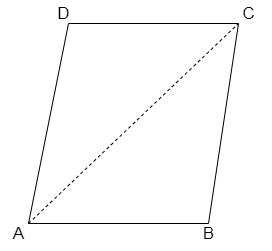• Draw AB = 4.5 cm.
• Taking B as centre, draw an arc of radius 5.5 cm.
• Now taking A as the centre, draw an arc of radius 7 cm intersecting with the arc made previously with centre B. Mark the intersection point as point C. Join AC and BC, successively.
• Taking A as the centre again, draw an arc of radius 6 cm.
• Taking D as the centre (which was marked previously), draw an arc of radius 4 cm, now intersect both the arcs, one of radius 6 cm made from point A and the other made from point C, and mark the intersection point as D (update the rough point).
• Now join AD and CD.
• Hence we have the required quadrilateral ABCD.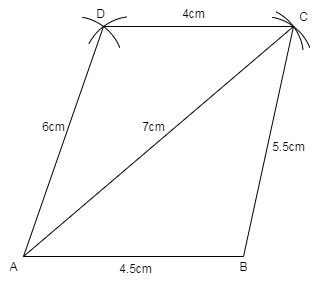### (ii) Quadrilateral JUMP       JU = 3.5 cm, UM = 4 cm, MP = 5 cm, PJ = 4.5 cm, PU = 6.5 cm

Procedure:

Given JU = 3.5 cm, UM = 4 cm, MP = 5 cm, PJ = 4.5 cm, PU = 6.5 cm
We have to construct a quadrilateral JUMP

• Draw JU = 3.5 cm.
• Taking J as centre draw an arc of radius 4.5 cm and taking U as centre draw an arc of 6.5 cm, such that both the arcs should intersect at point P. Join PJ and PU respectively.
• Similarly, taking P and U as centre draw arcs of 5 cm and 4 cm respectively, intersect both the arcs at M.
• Join MP and UM.
• Hence we have the required quadrilateral.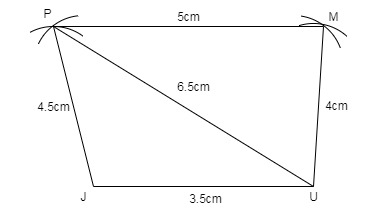### (iii) Parallelogram MORE        OR = 6 cm, RE = 4.5 cm, EO = 7.5 cm

Procedure:

Given OR = 6 cm, RE = 4.5 cm, EO = 7.5 cm
We have to construct a parallelogram MORE (Opposite sides are equal in a parallelogram).

• Draw an arc of 4.5 cm taking R as the centre.
• Draw an arc of 7.5 cm taking O as the centre, such that it intersects the previous arc.
• Join both the arcs at point E. Join EO and RE
• Taking E as centre draw an arc of 6 cm.
• Taking O as centre draw an arc of 4.5 cm.
• Intersect both the arcs at M. Join EM and OM.
• Therefore we have the required parallelogram MORE.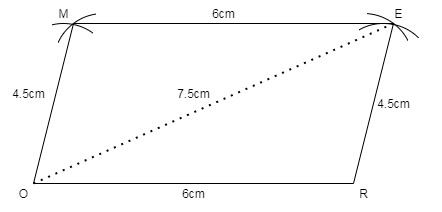### (iv) Rhombus BEST        BE = 4.5 cm, ET = 6 cm

Procedure:

Given BE = 4.5 cm, ET = 6 cm
We have to construct a Rhombus BEST (all sides of a rhombus are equal)

• Draw a triangle BET,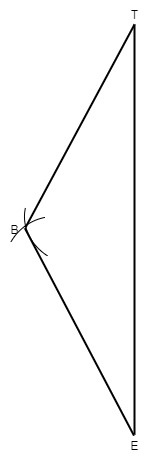• Draw ET = 6 cm.
• Draw an arc of 4.5 cm from both the points E and T respectively, join BE and BT.
• Similarly, taking E and T as centre draw arcs of 4.5 cm and intersect at S as shown below,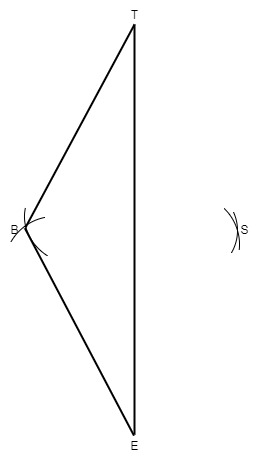• Join SE and ST.
• Join Diagonal BE. Measure it will be 4.5 cm.
• Therefore, we have the required Rhombus BEST.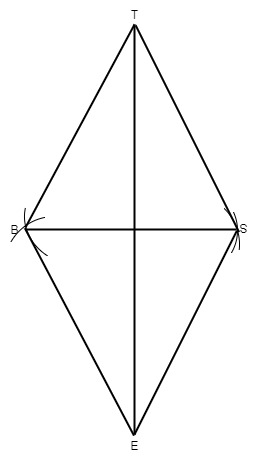My Personal Notes arrow_drop_up
Related Tutorials